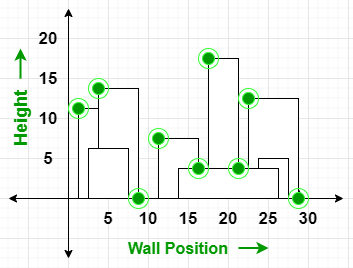# The Skyline Problem | Set 2

Given n rectangular buildings in a 2-dimensional city, computes the skyline of these buildings, eliminating hidden lines. The main task is to view buildings from aside and remove all sections that are not visible.
All buildings share common bottom and every building is represented by a triplet (left, ht, right)

• left: is x coordinated on the left side (or wall).
• right: is x coordinate of the right side.
• ht: is the height of the building.

A skyline is a collection of rectangular strips. A rectangular strip is represented as a pair (left, ht) where left is x coordinate of the left side of strip and ht is the height of strip.

Examples:

Input: buildings[][] = { {1, 11, 5}, {2, 6, 7}, {3, 13, 9}, {12, 7, 16}, {14, 3, 25}, {19, 18, 22}, {23, 13, 29}, {24, 4, 28} }
Output: { {1, 11}, {3, 13}, {9, 0}, {12, 7}, {16, 3}, {19, 18}, {22, 3}, {23, 13}, {29, 0} }
Explanation:
The skyline is formed based on the key-points (representing by “green” dots)
eliminating hidden walls of the buildings.Input: buildings[ ][ ] = { {1, 11, 5} }
Output: { {1, 11}, {5, 0} }

Approach:

1. From the given triplets for each building, retrieve the left wall location, height and right wall location value.
2. Store the left wall with its negative value of height and the right wall with its actual height as a pair in a vector walls. This is done in order to distinguish between left and right walls of the same building.
3. Sort the walls in ascending order.
4. Traverse the vector walls, if a left wall is found, store the height of the left wall in the multiset M. Otherwise, if a right wall is encountered, remove its corresponding height from the multiset.
5. Check if the top value has changed or not. If it has changed, then update the top value and store the current wall’s abscissa(x-cordinate) value and the updated top value in a vector as skyline.
6. Print the value pairs stored in the skyline vector.

Below is the implementation of the above approach:

## C++

 `// C++ progrqam for the above approach ` `#include ` `using` `namespace` `std; ` ` `  `// Function to create skyline ` `vector > ` `createSkyline(vector >& buildings) ` `{ ` ` `  `    ``// Get the number of buildings ` `    ``int` `N = buildings.size(); ` ` `  `    ``// To store the left and right ` `    ``// wall position of the buildings ` `    ``vector > wall; ` ` `  `    ``// Triplet of building structure ` `    ``// parameters ` `    ``int` `left, height, right; ` `    ``for` `(``int` `i = 0; i < N; i++) { ` ` `  `        ``// Get left point of building ` `        ``left = buildings[i]; ` ` `  `        ``// Get height of building ` `        ``height = buildings[i]; ` ` `  `        ``// Get right point of building ` `        ``right = buildings[i]; ` ` `  `        ``// Store left point and height ` `        ``// of the left wall ` ` `  `        ``// Negative value means left wall ` `        ``// will be inserted to multiset first ` `        ``// for the same abscissa(x) as right wall ` `        ``wall.push_back({ left, -height }); ` ` `  `        ``// Store right point and height ` `        ``// of the right wall ` `        ``wall.push_back( ` `            ``make_pair(right, height)); ` `    ``} ` ` `  `    ``// Sort the walls in ascending order ` `    ``sort(wall.begin(), wall.end()); ` ` `  `    ``// To store skyline: output ` `    ``vector > skyline; ` ` `  `    ``// Initialize a multiset to ` `    ``// keep left wall heights sorted ` `    ``multiset<``int``> leftWallHeight = { 0 }; ` ` `  `    ``// Current max height among ` `    ``// leftWallHeights ` `    ``int` `top = 0; ` ` `  `    ``// Traverse through the sorted walls ` `    ``for` `(``auto` `w : wall) { ` ` `  `        ``// If left wall is found ` `        ``if` `(w.second < 0) { ` ` `  `            ``// Insert the height ` `            ``leftWallHeight.insert(-w.second); ` `        ``} ` ` `  `        ``// If right wall is found ` `        ``else` `{ ` ` `  `            ``// Remove the height ` `            ``leftWallHeight.erase( ` `                ``leftWallHeight.find(w.second)); ` `        ``} ` ` `  `        ``// Mark a skyline point if top changes ` `        ``// .rbegin(): reverse iterator ` `        ``if` `(*leftWallHeight.rbegin() != top) { ` ` `  `            ``top = *leftWallHeight.rbegin(); ` `            ``skyline.push_back( ` `                ``make_pair(w.first, top)); ` `        ``} ` `    ``} ` ` `  `    ``// Return skyline to printSkyline ` `    ``return` `skyline; ` `} ` ` `  `// Function to print the output skyline ` `void` `printSkyline( ` `    ``vector >& buildings) ` `{ ` ` `  `    ``// Function call for creating skyline ` `    ``vector > skyline ` `        ``= createSkyline(buildings); ` ` `  `    ``cout << ``"Skyline for given"` `         ``<< ``" buildings:\n{"``; ` ` `  `    ``for` `(``auto` `it : skyline) { ` ` `  `        ``cout << ``"{"` `<< it.first << ``", "` `             ``<< it.second << ``"} "``; ` `    ``} ` `    ``cout << ``"}"``; ` `} ` ` `  `// Driver Code ` `int` `main() ` `{ ` `    ``vector > buildings;blockquote ` ` `  `    ``// Given left and right location ` `    ``// and height of the wall ` `    ``buildings = { { 1, 11, 5 }, { 2, 6, 7 },  ` `                  ``{ 3, 13, 9 }, { 12, 7, 16 }, ` `                  ``{ 14, 3, 25 }, { 19, 18, 22 }, ` `                  ``{ 23, 13, 29 }, { 24, 4, 28 } }; ` ` `  `    ``// Function Call ` `    ``printSkyline(buildings); ` `    ``return` `0; ` `} `

Output:

Skyline for given buildings:
{{1, 11} {3, 13} {9, 0} {12, 7} {16, 3} {19, 18} {22, 3} {23, 13} {29, 0} }

Time Complexity: O(N * log(N))
Auxiliary Space: O(N)My Personal Notes arrow_drop_upCheck out this Author's contributed articles.

If you like GeeksforGeeks and would like to contribute, you can also write an article using contribute.geeksforgeeks.org or mail your article to contribute@geeksforgeeks.org. See your article appearing on the GeeksforGeeks main page and help other Geeks.

Please Improve this article if you find anything incorrect by clicking on the "Improve Article" button below.